# DAV Class 5 Maths Chapter 6 Brain Teasers Solutions

The DAV Maths Book Class 5 Solutions and DAV Class 5 Maths Chapter 6 Brain Teasers Solutions of Addition and Subtraction of Decimal Numbers offer comprehensive answers to textbook questions.

## DAV Class 5 Maths Ch 6 Brain Teasers Solutions

Question 1.
(a) How much is 40 more than 12.25?
(i) 27.75
(ii) 28.25
(iii) 27.25
(iv) 28.75
Solution:
We have to subtract to compare the decimal numberSo, option (i) is correct.

(b) The digit in the hundredths place in the sum of 3.42, 6.2, and 2.473 is-
(i) 3
(ii) 0
(iii) 2
(iv) 9
Solution:
First, find the sum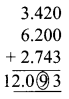So, option (iv) is correct.

(c) 109.93 + _____ = 349.109
(i) 239.179
(ii) 240.179
(iii) 249.279
(iv) 239.279
Solution:
We have to subtract.So, option (i) is correct(d) The difference between the greatest and the smallest numbers in the given set of numbers is-
7.018, 7.108, 7.810, 7.081
(i) 0.090
(ii) 0.792
(iii) 0.729
(iv) 0.027
Solution:
Greatest number = 7.810
Smallest number = 7.018
Difference = 0.792
option (ii) is correct.

(e) 0.1 + 0.001 – 0.01 = _____
(i) 0.091
(ii) 0.910
(iii) 0.901
(iv) 0.019
Solution: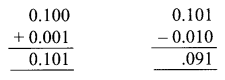option (i) is correct

Question 2.
Find the sum or difference of the following:
(a) 111.1 + 11.11 + 1.111
Solution:(b) 200.8 – 178.865
Solution:(c) 43 – 28.625
Solution: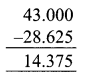(d) 85 + 8.583 + 85.1
Solution: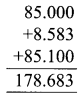Question 3.
The sum of two decimal numbers is 0.9. If one of them is 0.675, find the others.
Solution:
To find the other, we have to subtract it.
The sum of two decimal numbers = 0.900
One of the Numbers = -0.675
Another number = 0.225
Another number is 0.225

Question 4.
A tower is painted in red, white, and black. If 25.5 m is painted red, 15.75 m in black, and 10.25 in white, find the height of the tower.
Solution:
To find the height of the tower, we have to add all the portions.
Length of tower painted red = 25.50
Length of tower painted black = 15.75
Length of tower painted white = +10.25
Height of tower = 51.50
The height of the tower is 51.50 m

Question 5.
The sum of three decimal numbers is 95.3. If two decimal numbers are 43.82 and 26.058 respectively, find the third number.
Solution:
We have to subtract it, the sum of two decimal numbers from the sum of three decimal numbers.
One decimal number = 43.820
Other decimal number = +26.058
The sum of two decimal numbers = 69.878
The sum of three decimal numbers = 95.300
The sum of two decimal numbers = -69.878
Third number = 25.422
The third decimal number is 25.422Question 6.
Complete the following magic square so that the decimal numbers from left to right and top to bottom add upto to 4.5.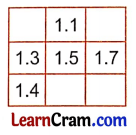Solution:
IInd row = 1.3 + 1.5 + 1.7 = 4.5
Ist column r1 = 4.5 – (1.3 + 1.4)
= 4.5 – 2.7
= 1.8
For column r2 = 4.5 – (1.1 + 1.5)
= 4.5 – 2.6
= 1.9
For row r3 = 4.5 – (1.8 + 1.1)
= 4.5 – 2.9
= 1.6
For column r4 = 4.5 – (1.6 + 1.7)
= 4.5 – 3.3
= 1.2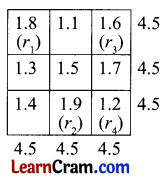Question 1.
(a)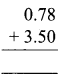Solution:
4.28

(b)Solution:
3.914(c)Solution:
219.86

(d)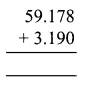Solution:
62.368

Question 2.
(a) 3.6 + 5.776 + 0.36
Solution:(b) 191.3 + 0.236 + 1.133
Solution: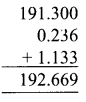(c) 12.805 + 7.37 + 0.133
Solution: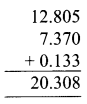Question 3.
Subtract.
(a) 7.30 from 9.135
Solution:(b) 18.355 from 20.13
Solution: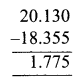(c) 91.013 from 103.56
Solution: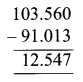(d) 33.12 from 90
Solution:Question 4.
Mr Sahni spent ₹ 50 on shaving, ₹ 90.50 on repairing shoes, and ₹ 120.75 on fruits. Find the total amount he spent.
Solution:
Money spent on shaving = ₹ 50.00
Money spent on repairing shoes = ₹ 90.50
Money spent on fruits = + ₹ 120.75
Amount he spent = ₹ 261.25
The total money he spent is ₹ 261.25.Question 5.
Fill in the blanks.
(a) 123.50 – _____ = 123.50
Solution:
0

(b) 56.25 – _____ = 0
Solution:
56.25

(c) 430.30 – _____ = 430.30
Solution:
0

(d) 76.361 – _____ = 0
Solution:
76.361

(e) 96.3 – 0 = _____
Solution:
96.3

(f) 79.89 – _____ = 0
Solution:
79.89

Question 6.
(a) How much is 80 more than 39.156?
(i) 40.344
(ii) 40.844
(iii) 40.130
(iv) 40.220
Solution:
(ii) 40.844

(i) 1.439
(ii) 1.379
(iii) 1.339
(iv) 1.439
Solution:
(iii) 1.339(c) 0.624 + 1.234 + 1.8 – 2.555
(i) 1.35
(ii) 1.39
(iii) 0.955
(iv) 1.103
Solution:
(iv) 1.103

Question 7.
Write the digit in the tenths place in the sum of 7.351, 9.253, and 3.56.
Solution:
1

Question 8.
The sum of the two numbers is 107.36. If one of the numbers is 36.7. Find the other number.
Solution:
The sum of two numbers = 107.36
One of the numbers = 36.7
other number = 70.66

Question 9.
Solve
(a) 12.480 + 13.990 – 10
Solution:
16.470

(b) 48.620 + 28.03 – 33.006
Solution:
43.644

(c) 156 + 65.3 – 200.008
Solution:
21.292

(d) 137.12 + 329.1 – 222.463
Solution:
243.757Question 10.
The length of a wall painted black and white is 156.360 m. 90.76 m is painted black. Find the portion painted white.
Solution:
Length of a wall painted black and white = 156.360 m
Length of a wall painted black = 90.76 m
Length of a wall painted white = 65.6 m

Question 11.
The sum of three decimal numbers is 115.78. If two decimal numbers are 20.368 and 56.12 respectively. Find the third number.
Solution:
The sum of three decimal numbers = 115.78
Sum of two decimal numbers = 20.368 + 56.12 = 76.488
the third number = 39.292